TitleCollege Algebra
Answer/Discussion to Practice Problems
Tutorial 11: Complex Rational Expres
sions

WTAMU > Virtual Math Lab > College Algebra > Tutorial 11: Complex Rational ExpressionsAnswer/Discussion to 1a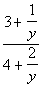Step 1:   If needed, rewrite the numerator and denominator so that they are each a single fraction.

 Combining only the numerator we get: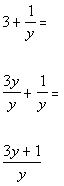*Rewrite fractions with LCD of y

 Combining only the denominator we get: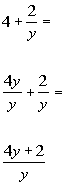*Rewrite fractions with LCD of y

 Putting these back into the complex fraction we get: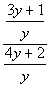*Write numerator over denominator

 AND Step 3: If needed, simplify the rational expression.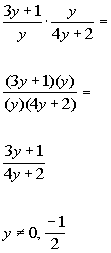*Rewrite div. as mult. of reciprocal     *Divide out a common factor of y         *Excluded values of the original den.

 Note that the values that would be excluded from the domain are 0 and -1/2.  These are the values that make the original denominators equal to 0.Answer/Discussion to 1b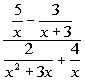Step 1: Multiply the numerator and denominator of the overall complex fractions by the LCD of the smaller fractions.

 The denominators of the numerator's fractions have the following factors: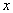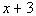The denominators of the denominator's fractions  have the following factors: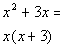Putting all the different factors together and using the highest exponent, we get the following LCD for all the small fractions: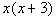Multiplying numerator and denominator by the LCD we get: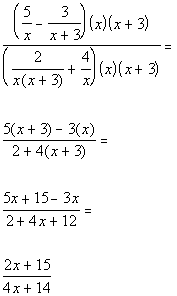*Mult. num. and den. by x(x +3)

 Step 2: If needed, simplify the rational expression.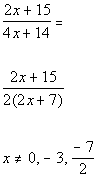*Factor the GCF of 2 *No common factors to divide out   *Excluded values of the original den.

 Note that the values that would be excluded from the domain are 0, -3 and -7/2.  These are the values that make the original denominators equal to 0.

WTAMU > Virtual Math Lab > College Algebra > Tutorial 11: Complex Rational Expressions

Last revised on Dec. 15, 2009 by Kim Seward.
All contents copyright (C) 2002 - 2010, WTAMU and Kim Seward. All rights reserved.